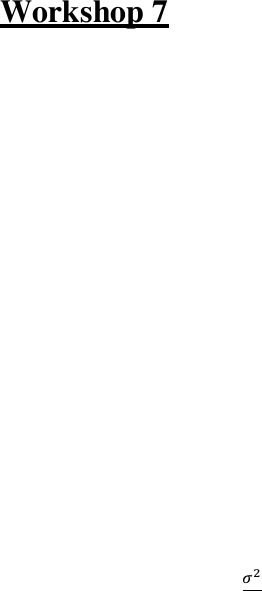# BUSS1020 Study Guide - Midterm Guide: Sample Space, Standard Deviation, Point Estimation

47 views7 pagesWorkshop 7
Samples from a Population, Shape of Sampling Distribution
Learning Objectives:
After completion of this workshop, students should be able to:
1. Enumerate the sample space for the sample mean, from samples of size 2 or 3 from a discrete
distribution.
2. Determine the sampling distribution of the mean, after enumerating the sample space.
3. Calculate the mean and variance of the sampling distribution of the mean.
4. Calculate a point estimate for the mean and variance; be able to explain the meaning of a point
estimate, and what it is estimating.
5. Use Excel to create a set of random numbers to enable taking a sample.
6. Use the random number generator in Excel to produce random numbers from a normal or uniform
distribution.
7. Demonstrate, using expectations, that 
  and
 
, for random samples
8. Choose the distribution to be used and explain why the choice was made.
9. Understand when the Central Limit Theorem (CLT) is used and how to use it.
10. Calculate an interval estimation for the population mean, when the population standard deviation
is known, using the CLT.
11. State the assumptions for the choice of distribution when calculating a confidence interval estimate
for the population mean.
12. Interpret the confidence interval estimate obtained for the population mean.
Unlock document

This preview shows pages 1-2 of the document.
Unlock all 7 pages and 3 million more documents.Learning the Basics
(These questions are to be done before the workshop. They will be corrected at the start of the
workshop.)
Be prepared to give answers to these questions in the workshop.
Question 1 Consider a population consisting of the measurements, 0, 4 and 12 and described by the
probability distribution below.
X
0
4
12
P(x)
0.3
0.2
0.5
A random sample of n=2 measurements is selected from the population (with replacement).
In table format,
(a) Write down all possible samples.
(b) Calculate the probability for each possible sample. Check that this is a proper probability
distribution.
(c) Calculate the mean for each possible sample.
(d) Summarise and graph the values of the mean together with their respective probabilities. This is
the probability (or sampling) distribution of the mean.
(e) Find E(X) = P, V(X) = V2. Check that 
  and
 
hold in this case.
Question 2
Question 1, page 277 in the textbook
Question 3
Question 15, page 281 in the textbook.
Question 4
Question 16, page 281 in the textbook.
Question 5
Question 23, page 283 in the textbook. You may like to open up STATCRUNCH, go to Applets and click on
of p (say 0.01, 0.05, 0.15, 0.25, 0.5) and n (say 2, 5 10, 25, 50, 100, 1000). Keep clicking the “1000 times”
button to increase the simulation sample size.
Question 6
Question 25, page 283 in the textbook.
Unlock document

This preview shows pages 1-2 of the document.
Unlock all 7 pages and 3 million more documents.